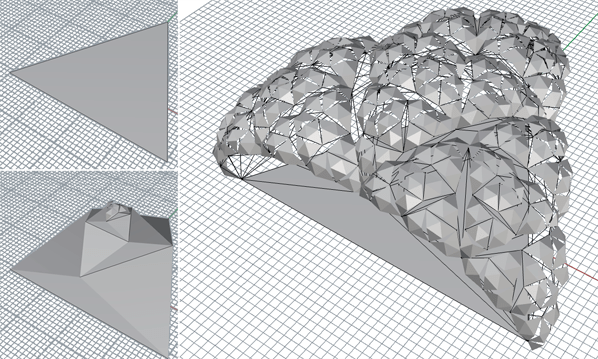##### Archiologics

Studio archiologics is a design think tank moved by the need of innovative thinking in architecture. We believe that complex, integrative thinking leads to a complex architecture which can be solved through simple methodologies and analytic algorithmic procedures.# Recursive triangulation in RhinoPython## 17 Jul Recursive triangulation in RhinoPython

This recursive scripts generates a tree-like structure based on a simple algorithm. Each branch creates four sub-branches in the next level, making it very easy to multiply geometry in an exponential manner. Be careful as it does not provide sufficient error-checking, it simply assumes that you input the correct geometry.

#brocoli subdivision

#import libraries

import random

tmpSrfList = []

#define our function

def subdivideTriaSurf(srf,delete,scFactor):

#duplicate border->returns a list

#in this case we know we only want to have the first object 

polyBorder = polyBorderList

#test

print (polyBorder)

#get segments from the border as individual curves (helps get the middle points)

segment1 = segments

segment2 = segments

segment3 = segments

#calculate middle points

#calculate vertex

pt1 = vertexPts

pt2 = vertexPts

pt3 = vertexPts

#calculate normal vector

#since normal vector is BY DEFAULT 0-based, we add it to the midPoints

#to obtain the normals

“””

“””

if(len>5):

subdivideTriaSurf(baseSrf,delete,scFactor)

subdivideTriaSurf(triaSrf1,delete,scFactor)

subdivideTriaSurf(triaSrf2,delete,scFactor)

subdivideTriaSurf(triaSrf3,delete,scFactor)

tmpSrfList.append(baseSrf)

tmpSrfList.append(triaSrf1)

tmpSrfList.append(triaSrf2)

tmpSrfList.append(triaSrf3)

if(delete==”Yes”):

#obtain geometry

userSrf = rss.GetObject(“Please select your surface AND make sure that it needs to be defined by 3 points”,8)

#obtain other variables

userDelete = rss.GetString(“Do you want to delete the temp surfaces?, you can alway s do it later”,None,(“Yes”,”No”))

print userDelete

#call the function

subdivideTriaSurf(userSrf,userDelete,scaleFactor)

#ask the user to erase temp surfaces

if (userDelete==”No”):

userDeleteAfter = rss.GetString(“Do you want to delete the temp surfaces?”,None,(“Yes”,”No”))

if(userDeleteAfter ==”Yes”):